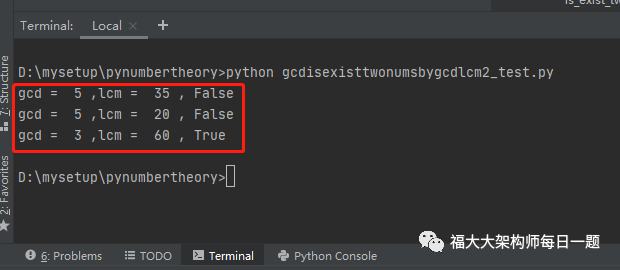# 用python判断两个数的最大公约数_20200922：已知两个数的最大公约数和最小公倍数，并且这两个数不能是最大公约数和最小公倍数本身。如何判断这两个数是否存在...

2020-09-22：已知两个数的最大公约数和最小公倍数，并且这两个数不能是最大公约数和最小公倍数本身。如何判断这两个数是否存在？

1.如果最小公倍数不能被最大公约数整除，不存在这两个数。

2.求【商】=【最小公倍数/最大公约数】。

3.判断【商】是否是质数，如果是，直接返回false。这个步骤可以不要。

4.幂次方缩小【商】范围，如果【商】是a的b次方，【商】变成a。

5.判断【商】是否是质数，如果是，直接返回false。

6.经过所有考验，返回true。

# -*-coding:utf-8-*-import math# 求快速幂。ret = a^b%p。def quick_power(a, b, p):    """    求快速幂。ret = a^b%p。    Args:        a: 底数。大于等于0并且是整数。        b: 指数。大于等于0并且是整数。        p: 模数。大于0并且是整数。    Returns:        返回结果。    Raises:        IOError: 无错误。    """    a = a % p    ans = 1    while b != 0:        if b & 1:            ans = (ans * a) % p        b >>= 1        a = (a * a) % p    return ans# 求num的exp开方,exp是指数，num是结果。求底数。def _get_sqrt_range(num, right, exp=2):    """        求num的exp开方,exp是指数，num是结果。求底数。        Args:            num: 大于等于0并且是整数。            right: 大于等于0并且是整数。右边界。            exp: 大于等于0并且是整数。        Returns:            返回元组，表示一个开方范围。        Raises:            IOError: 无错误。    """    left = 1    if num == 0:        return 0, 0    if num == 1:        return 1, 1    if num == 2 or num == 3:        return 1, 2    while True:        mid = (left + right) // 2        if mid ** exp > num:            right = mid            if left ** exp == num:                return left, left            if left + 1 == right:                return left, right        elif mid ** exp < num:            left = mid            if right ** exp == num:                return right, right            if left + 1 == right:                return left, right            if mid == 1:                return 1, 2        else:            return mid, mid# 求对数范围def get_log_range(num, basenum):    """        求对数范围。        Args:            num: 数，大于等于1并且是整数。            basenum: 底数，大于等于2并且是整数。        Returns:            返回结果。对数范围。        Raises:            IOError: 无错误。    """    if num == 1:        return 0, 0    else:        n = 0        ism = 0        while num >= basenum:            if ism == 0 and num % basenum != 0:                ism = 1            n += 1            num //= basenum        return n, n + ism# 判断幂次方，并且返回底数def is_power2(num):    """        判断n是否是一个数的幂次方形式。        Args:            num: 大于等于0并且是整数。        Returns:            返回结果。true是幂数        Raises:            IOError: 无错误。    """    if num <= 3:        return False, 0    else:        log_range = get_log_range(num, 2)        if log_range == log_range:            return True, 2        expmax = log_range        expmin = 2        exp = expmin        sqrt = 0        right = 2 ** (1 + log_range // 2)        while exp <= expmax:            sqrt = _get_sqrt_range(num, right, exp)            right = sqrt  # 缩小右边界范围            if sqrt == sqrt:                return True, sqrt            if sqrt == (1, 2):                return False, 0            exp += 1        return False, 0# 米勒-拉宾素性检验是一种概率算法，但是，Jim Sinclair发现了一组数：2, 325, 9375, 28178, 450775, 9780504, 1795265022。用它们做 [公式] ， [公式] 以内不会出错，我们使用这组数，就不用担心运气太差了。def is_prime_miller_rabin(num):    """        判断是否是素数。米勒拉宾素性检验是一种概率算法 可能会把合数误判为质数。        Args:            num: 大于等于2并且是整数。        Returns:            返回结果。true为素数；false是非素数。        Raises:            IOError: 无错误。    """    # num=(2^s)*t    a = 2  # 2, 325, 9375, 28178, 450775, 9780504, 1795265022    s = 0    t = num - 1    num_1 = t    if num == 2:        return True    if not (num % 2):        return False    while not (t & 1):        t >>= 1        s += 1    k = quick_power(a, t, num)    if k == 1:        return True    j = 0    while j < s:        if k == num_1:            return True        j += 1        k = k * k % num    return False# 综合法def is_prime_comprehensive(num):    """        判断是否是素数。综合算法:试除法+米勒拉宾素性检验 可能会把合数误判为质数。        Args:            num: 大于等于2并且是整数。        Returns:            返回结果。true为素数；false是非素数。        Raises:            IOError: 无错误。    """    if num <= 1:        return False    if num == 2:        return True    if num & 1 == 0:        return False    # 100以内的质数表    primeList = [3, 5, 7, 11, 13, 17, 19, 23, 29, 31, 37, 41, 43, 47, 53, 59, 61, 67, 71, 73, 79, 83, 89, 97]    # 质数表是否能整除    for prime in primeList:        if num == prime:            return True        if num % prime:            if prime * prime >= num:                return True        else:            return False    # 米勒拉宾素性检验    return is_prime_miller_rabin(num)# 已知两个数的最大公约数和最小公倍数，并且这两个数不能是最大公约数和最小公倍数本身。如何判断这两个数是否存在？def is_exist_two_nums_by_gcd_lcm_not(gcd, lcm):    """        已知两个数的最大公约数和最小公倍数，并且这两个数不能是最大公约数和最小公倍数本身。如何判断这两个数是否存在？        Args:            gcd: 大于等于1并且是整数。最大公约数。            lcm: 大于等于1并且是整数。最小公倍数。        Returns:            返回True，说明存在。        Raises:            IOError: 无错误。    """    # 1.如果最小公倍数不能被最大公约数整除，不存在这两个数。    if lcm % gcd != 0:        return False    # 2.求【商】=【最小公倍数/最大公约数】。    quotient = lcm // gcd    # 3.判断【商】是否是质数，如果是，直接返回false。这个步骤可以不要。    if is_prime_comprehensive(quotient):        return False    # 4.幂次方缩小【商】范围，如果【商】是a的b次方，【商】变成a。    isloop = True    quotienttemp = 0    while isloop:        isloop, quotienttemp = is_power2(quotient)        if isloop:            quotient = quotienttemp    # 5.判断【商】是否是质数，如果是，直接返回false。    if is_prime_comprehensive(quotient):        return False    # 6.经过所有考验，返回true。    return Trueif __name__ == "__main__":    gcd = 5    lcm = 35    print("gcd = ", gcd, ",lcm = ", lcm, ",", is_exist_two_nums_by_gcd_lcm_not(gcd, lcm))    gcd = 5    lcm = 20    print("gcd = ", gcd, ",lcm = ", lcm, ",", is_exist_two_nums_by_gcd_lcm_not(gcd, lcm))    gcd = 3    lcm = 60    print("gcd = ", gcd, ",lcm = ", lcm, ",", is_exist_two_nums_by_gcd_lcm_not(gcd, lcm))***

[评论](https://user.qzone.qq.com/3182319461/blog/1600735568)

09-083万+01-229029
11-30875
10-171613
12-083710
03-226万+
11-127251
03-311万+
04-27294
01-14143
07-252万+
03-311万+
01-24200
11-052万+
10-29630
03-094万+
03-09685
10-072万+
©️2020 CSDN 皮肤主题: 游动-白 设计师:白松林